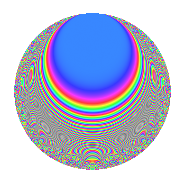# Properties

 Label 287.2.h.cLevel 287 Weight 2 Character orbit 287.h Analytic conductor 2.292 Analytic rank 0 Dimension 40 CM No

# Related objects

## Newspace parameters

 Level: $$N$$ = $$287 = 7 \cdot 41$$ Weight: $$k$$ = $$2$$ Character orbit: $$[\chi]$$ = 287.h (of order $$5$$ and degree $$4$$)

## Newform invariants

 Self dual: No Analytic conductor: $$2.29170653801$$ Analytic rank: $$0$$ Dimension: $$40$$ Relative dimension: $$10$$ over $$\Q(\zeta_{5})$$ Sato-Tate group: $\mathrm{SU}(2)[C_{5}]$

## $q$-expansion

The dimension is sufficiently large that we do not compute an algebraic $$q$$-expansion, but we have computed the trace expansion.

 $$\operatorname{Tr}(f)(q) =$$ $$40q - 3q^{2} - 3q^{4} - 4q^{5} - 19q^{6} - 10q^{7} + 16q^{8} + 60q^{9} + O(q^{10})$$ $$\operatorname{Tr}(f)(q) =$$ $$40q - 3q^{2} - 3q^{4} - 4q^{5} - 19q^{6} - 10q^{7} + 16q^{8} + 60q^{9} + 5q^{10} - 10q^{11} - 12q^{12} - 17q^{13} + 2q^{14} - 3q^{15} - 23q^{16} - 8q^{17} - 2q^{18} + 23q^{19} - 13q^{22} - q^{23} + 46q^{24} - 34q^{25} + 3q^{26} - 18q^{28} - 18q^{29} - 19q^{30} - 3q^{31} - 26q^{32} - 6q^{33} - 44q^{34} + q^{35} - 38q^{36} - 5q^{37} + 28q^{38} + 17q^{39} + 14q^{40} - 11q^{41} - 24q^{42} + 13q^{43} + 66q^{44} + 43q^{45} - 20q^{46} - 27q^{47} + 39q^{48} - 10q^{49} + 106q^{50} - 18q^{51} - 30q^{52} - 30q^{53} - 109q^{54} + 118q^{55} + 16q^{56} - 40q^{57} - 23q^{58} - 37q^{59} + 96q^{60} - 41q^{61} - 13q^{62} - 30q^{63} + 10q^{64} + 6q^{65} - 30q^{66} - 6q^{67} - 26q^{68} - 31q^{69} + 5q^{70} - 31q^{71} + 107q^{72} - 46q^{73} + 75q^{74} - 61q^{75} + 43q^{76} - 10q^{77} + 34q^{78} + 76q^{79} + 64q^{80} + 16q^{81} - 16q^{82} - 52q^{83} - 7q^{84} + 86q^{85} - 17q^{86} - 20q^{87} - 52q^{88} - 16q^{89} + 6q^{90} + 18q^{91} + 97q^{92} + 32q^{93} - 5q^{94} - 102q^{95} + 38q^{96} - 18q^{97} - 3q^{98} - 71q^{99} + O(q^{100})$$

## Embeddings

For each embedding $$\iota_m$$ of the coefficient field, the values $$\iota_m(a_n)$$ are shown below.

For more information on an embedded modular form you can click on its label.

Label $$a_{2}$$ $$a_{3}$$ $$a_{4}$$ $$a_{5}$$ $$a_{6}$$ $$a_{7}$$ $$a_{8}$$ $$a_{9}$$ $$a_{10}$$
57.1 −0.794058 + 2.44386i 3.29652 −3.72389 2.70556i 0.799736 + 0.581042i −2.61763 + 8.05622i 0.309017 + 0.951057i 5.41126 3.93151i 7.86702 −2.05502 + 1.49306i
57.2 −0.662436 + 2.03877i 0.259063 −2.09972 1.52553i 0.0549948 + 0.0399561i −0.171613 + 0.528169i 0.309017 + 0.951057i 1.03258 0.750212i −2.93289 −0.117892 + 0.0856533i
57.3 −0.508764 + 1.56581i −0.349538 −0.574899 0.417688i −3.08337 2.24020i 0.177832 0.547311i 0.309017 + 0.951057i −1.71741 + 1.24777i −2.87782 5.07644 3.68825i
57.4 −0.279797 + 0.861126i 2.59818 0.954783 + 0.693690i −1.43627 1.04351i −0.726962 + 2.23736i 0.309017 + 0.951057i −2.32953 + 1.69251i 3.75053 1.30046 0.944836i
57.5 −0.149394 + 0.459786i −1.52804 1.42895 + 1.03819i 1.42271 + 1.03366i 0.228280 0.702574i 0.309017 + 0.951057i −1.47306 + 1.07024i −0.665079 −0.687806 + 0.499720i
57.6 0.223825 0.688863i 1.30681 1.19360 + 0.867201i 1.13385 + 0.823787i 0.292498 0.900216i 0.309017 + 0.951057i 2.03650 1.47961i −1.29224 0.821260 0.596680i
57.7 0.228466 0.703146i −2.48892 1.17582 + 0.854281i −2.55629 1.85725i −0.568634 + 1.75007i 0.309017 + 0.951057i 2.06558 1.50073i 3.19473 −1.88994 + 1.37312i
57.8 0.450739 1.38723i 0.560317 −0.103210 0.0749862i 0.738519 + 0.536566i 0.252556 0.777289i 0.309017 + 0.951057i 2.20955 1.60533i −2.68604 1.07722 0.782646i
57.9 0.571535 1.75900i −3.10319 −1.14941 0.835096i 2.72110 + 1.97700i −1.77358 + 5.45852i 0.309017 + 0.951057i 0.866730 0.629716i 6.62977 5.03276 3.65651i
57.10 0.728900 2.24332i −0.551198 −2.88317 2.09475i −1.91302 1.38989i −0.401768 + 1.23652i 0.309017 + 0.951057i −2.98417 + 2.16813i −2.69618 −4.51238 + 3.27844i
78.1 −2.17748 + 1.58203i 2.12926 1.62055 4.98755i −1.11426 + 3.42935i −4.63642 + 3.36856i −0.809017 0.587785i 2.69829 + 8.30447i 1.53376 −2.99905 9.23012i
78.2 −1.91136 + 1.38868i −1.05524 1.10682 3.40644i 0.886655 2.72884i 2.01694 1.46539i −0.809017 0.587785i 1.15479 + 3.55408i −1.88648 2.09479 + 6.44709i
78.3 −1.52332 + 1.10676i 2.82445 0.477560 1.46978i 0.756805 2.32921i −4.30254 + 3.12598i −0.809017 0.587785i −0.264503 0.814055i 4.97750 1.42501 + 4.38572i
78.4 −1.49937 + 1.08936i −1.69208 0.443381 1.36459i −0.353412 + 1.08769i 2.53705 1.84327i −0.809017 0.587785i −0.323689 0.996211i −0.136877 −0.654987 2.01584i
78.5 −0.224492 + 0.163103i −2.77195 −0.594240 + 1.82888i −1.20463 + 3.70748i 0.622280 0.452113i −0.809017 0.587785i −0.336391 1.03531i 4.68369 −0.334271 1.02878i
78.6 0.539909 0.392267i −3.00502 −0.480406 + 1.47854i 0.818883 2.52026i −1.62244 + 1.17877i −0.809017 0.587785i 0.733059 + 2.25612i 6.03012 −0.546493 1.68193i
78.7 0.635695 0.461859i 2.52804 −0.427240 + 1.31491i 0.910194 2.80129i 1.60706 1.16760i −0.809017 0.587785i 0.821337 + 2.52781i 3.39096 −0.715196 2.20115i
78.8 0.991525 0.720385i 2.24376 −0.153867 + 0.473553i −1.02540 + 3.15585i 2.22474 1.61637i −0.809017 0.587785i 0.946036 + 2.91160i 2.03444 1.25672 + 3.86779i
78.9 1.84039 1.33712i 1.18259 0.981102 3.01952i 0.156878 0.482822i 2.17642 1.58126i −0.809017 0.587785i −0.825922 2.54193i −1.60148 −0.356874 1.09834i
78.10 2.01949 1.46724i −2.38381 1.30749 4.02404i 0.286326 0.881220i −4.81408 + 3.49763i −0.809017 0.587785i −1.72104 5.29681i 2.68257 −0.714733 2.19972i
See all 40 embeddings
 $$n$$: e.g. 2-40 or 990-1000 Embeddings: e.g. 1-3 or 141.10 Significant digits: Format: Complex embeddings Normalized embeddings Satake parameters Satake angles

## Inner twists

This newform does not have CM; other inner twists have not been computed.

## Hecke kernels

This newform can be constructed as the kernel of the linear operator $$T_{2}^{40} + \cdots$$ acting on $$S_{2}^{\mathrm{new}}(287, [\chi])$$.